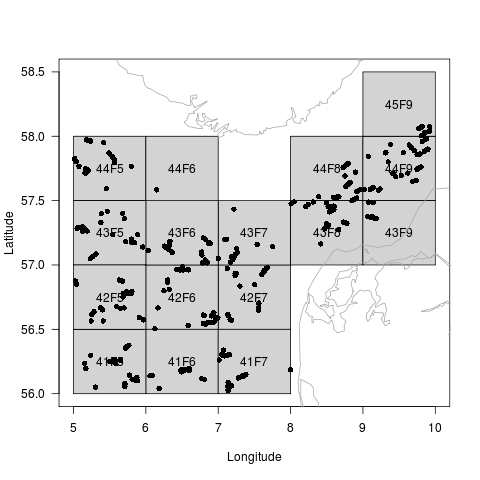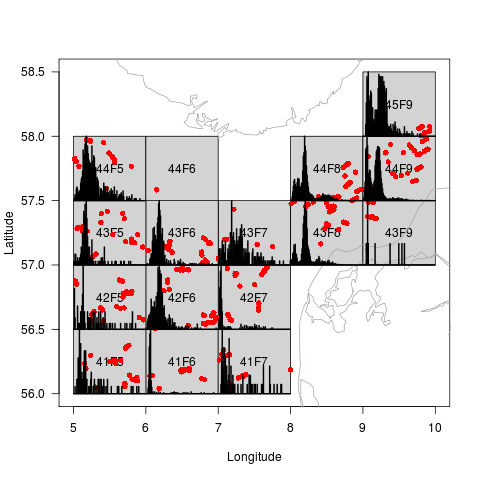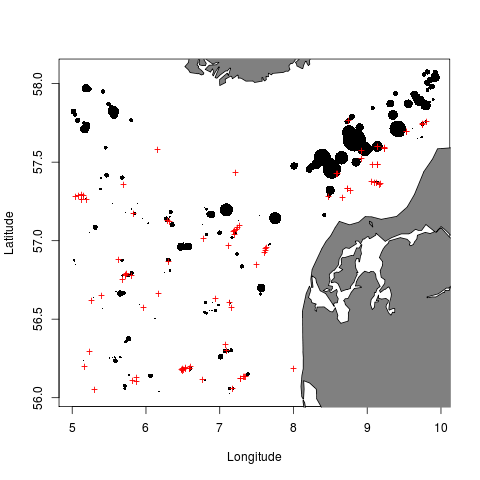DATRAS - The DATRAS library contains functions for reading and manipulating trawl survey data from the DATRAS database (datras.ices.dk)

Tutorial

# Tutorial

## 1 Introduction

Handling and interpreting data from DATRAS correctly from scratch takes a significant amount of effort and time, but this R package can reduce much of this workload to a few lines of code. The raw exchange format can be read into a `DATRASraw` object in `R` using the package. These data objects contain three components

1. Age data (CA records) - one vector per individual fish
2. Hydro data (HH records) - one vector per haul, position and experimental conditions.
3. Length data (HL records) - numbers per length group by haul and species

One particular useful function in the DATRAS package is the `subset()` function. It allows subsetting over all three components at once, without the need for specifying for which component(s) the subset clauses apply, because the function will look for the variable names in all components and apply the clauses where appropriate.

## 2 Getting started

### 2.1 Install the DATRAS package

To install the DATRAS package, start R and type

```install.packages('DATRAS',repos='http://www.rforge.net/',type='source')
```

Data can be downloaded from the web page http://datras.ices.dk/Data_products/Download/Download_Data_public.aspx . Make sure the Data products check box is set to Exchange Data. Also make sure that the check boxes HH, HL and CA are marked. In this example we will consider the survey NS-IBTS, with all quarters from 2006-2012 and all ships represented. Submit the query, and a file called Exchange will be downloaded. The data file used in this tutorial is also available here http://www.rforge.net/DATRAS/Exchange .

Advanced users can take advantage of a php script shipping with the DATRAS package. It requires command line php installed. For instance, to download data for the NS-IBTS survey for the period 1995-2005 run

```downloadExchange("NS-IBTS",1995:2005)
```

### 2.3 Read data into R

```library(DATRAS)
```

To see what is in the data object do

```print(d)
```
```Object of class 'DATRASraw'
===========================
Number of hauls: 5054
Number of species: 211
Number of countries: 8
Years: 2006 2007 2008 2009 2010 2011 2012
Quarters: 1 2 3
Gears: GOV
Haul duration: 0 - 58 minutes
```

### 2.4 What is in the `DATRASraw` object?

The individual components of a `DATRASraw` object are accessed using the double square bracket operator:

```names(d[["CA"]])
```
```  "RecordType"        "Survey"            "Quarter"
 "Country"           "Ship"              "Gear"
 "SweepLngt"         "GearExp"           "DoorType"
 "StNo"              "HaulNo"            "Year"
 "SpecCodeType"      "SpecCode"          "AreaType"
 "AreaCode"          "LngtCode"          "LngtClas"
 "Sex"               "Maturity"          "PlusGr"
 "Age"               "NoAtALK"           "IndWgt"
 "DateofCalculation" "StatRec"           "LngtCm"
 "Species"           "haul.id"
```

Beyond the default variables, the extra variables LngtCm, Species and haul.id have been added automatically.

Likewise , the hydro and length data records are accessed by

```names(d[["HH"]])
names(d[["HL"]])
```

For instance, to list the 10 most common occuring length groups by species run

```summary(d[["HL"]]\$Species)[1:10]
```
```        Merlangius merlangus              Limanda limanda
63383                        61977
Clupea harengus     Melanogrammus aeglefinus
48354                        46988
Pleuronectes platessa           Eutrigla gurnardus
41690                        40594
31214                        24598
Sprattus sprattus             Microstomus kitt
20650                        19603
```

### 2.5 Simple data subsetting

```dd <- subset(d,Species=="Gadus morhua",25<HaulDur & HaulDur<35, 5<lon & lon<10 & 56<lat & lat<60 )
```

The reduced data set contains

```print(dd)
```
```Object of class 'DATRASraw'
===========================
Number of hauls: 424
Number of species: 1
Number of countries: 7
Years: 2006 2007 2008 2009 2010 2011 2012
Quarters: 1 3
Gears: GOV
Haul duration: 27 - 32 minutes
```

### 2.6 What can be added to the object ?

For convenience, various types of derived quantities and meta-data can be added to the `DATRASraw` object. Most importantly, the raw number of observed individuals per length group per haul is added as follows

Size spectra on haul level are conveniently analysed using the `addSpectrum()` function, which adds the numbers caught per length group (cm) in the variable `N` on component 2. For example, to add the spectrum on data from Quarter 1 and list column 10 to 15 in the spectrum for the six first hauls:

```dQ1 <- subset(dd,Quarter==1)
```
```                           sizeGroup
haul.id                     [16,17) [17,18) [18,19) [19,20) [20,21) [21,22)
2006:1:DEN:DAN2:GOV:26:6        0       0       0       0       0       0
2006:1:DEN:DAN2:GOV:28:7        1       0       0       0       0       0
2006:1:DEN:DAN2:GOV:44:10       0       0       0       0       0       1
2006:1:DEN:DAN2:GOV:42:9        0       0       0       0       0       0
2006:1:DEN:DAN2:GOV:40:8        1       1       0       0       0       0
2006:1:DEN:DAN2:GOV:24:5        0       0       0       0       0       0
```

Further, numbers at age can be added by

```dQ1 <- addNage(dQ1)
```

see later details.

Spatial data from a shapefile can be added to the hydro data as follows

```dQ1 <- addSpatialData(dQ1,"ICES_areas.shp")
```

Shapefiles with ICES areas can be found at http://geo.ices.dk. This allows standard ICES areas to be extracted from a `DATRASraw` object:

```dQ1 <- subset(dQ1,ICES_area=="IIIa")
```

## 3 Visualizing data

### 3.1 Spatial plot

The default plot method of the DATRASraw object provides an overview of the trawl locations

```plot(dd)
```And if a length spectrum has been added, the length distribution is displayed for each ICES square:

```dd <- addSpectrum(dd)
plot(dd,col="red")
```### 3.2 Cohort plot

To see the temporal evolution of the length distribution of the stock the function plotVBG is useful

```plotVBG(dd,scale=2,ylim=c(0,60),col=c(2,4),lwd=2,by=paste(Year,Quarter))
```### 3.3 Biomass plot

To plot bubbles with areas proportional to the observed biomass in each haul, and red crosses for zero catch hauls, try

```dd <- addWeightByHaul(dd)
bubblePlot(dd)
```## 4 Age-Length keys

### 4.1 Simple continuation-ratio logits

Fit age-length key and predict numbers-at-age

```library(mgcv)
```
```alk <- fitALK(dQ1,minAge=1,maxAge=4)
dQ1\$Nage = predict(alk)
```
```                1            2            3           4+
[1,] 2.220446e-16 2.448222e-05 7.392315e-02 9.260524e-01
[2,] 1.992781e+00 7.218763e-03 2.278510e-07 4.058172e-11
[3,] 1.902358e+00 2.072981e+00 2.455574e-02 1.049175e-04
[4,] 0.000000e+00 0.000000e+00 0.000000e+00 0.000000e+00
[5,] 4.978305e+00 2.170079e-02 3.925881e-02 2.960736e+00
[6,] 0.000000e+00 0.000000e+00 0.000000e+00 0.000000e+00
```

Plot age length key (smooth and raw proportions by length group)

```plotALKfit(alk,row=1)